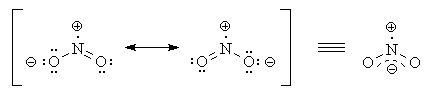# Which of the arrangements of Bond Order is correct for the following?

## $N {O}_{2}^{+} > N {O}_{2}^{-} > N {O}_{3}^{-}$ NO_3^-)>NO_2^+>NO_2^- NO_2^+=NO_2^-)>NO_3^- NO_2^-)>NO_2^+>NO_3^- Please explain in details.. Thank you!

Nov 4, 2016

The correct arrangement of bond orders is $\text{NO"_2^"+" > "NO"_2^"-" > "NO"_3^"-}$.

#### Explanation:

The bond order ($\text{BO}$) in a resonance hybrid depends on the average number of electrons shared between the atoms.

It takes two shared electrons to form a bond, so

$\text{BO" = "number of shared electrons"/"2 × number of bonds}$

$\boldsymbol{\text{NO"_2^"+}}$

The structure of the nitronium ion is

$\text{:Ö="stackrel(+)("N")"=Ö:}$

There are two $\text{N=O}$ double bonds (8 shared electrons).

$\text{BO" = "8 electrons"/"2 × 2 bonds} = 2$

Each bond is on average a double bond.

$\boldsymbol{\text{NO"_2^"-}}$

The structure of the nitrite ion isEach contributor to the resonance hybrid has an $\text{N=O}$ double bond and an $\text{N-O}$ single bond (6 shared electrons).

$\text{BO" = "6 electrons"/"2 × 2 bonds} = 1.5$

Each bond is on average a "1½ bond".

$\boldsymbol{\text{NO"_3^"-}}$

The three contributors to the resonance structure of nitrate ion areEach contributor to the resonance hybrid has an $\text{N=O}$ double bond and two $\text{N-O}$ single bonds (8 shared electrons).

$\text{BO" = "8 electrons"/"2 × 3 bonds} = 1.33$

Each bond is on average a "1⅓ bond".

∴ The correct arrangement of bond orders is $\text{NO"_2^"+" > "NO"_2^"-" > "NO"_3^"-}$.